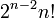# Perfect not implies semisimple

This article gives the statement and possibly, proof, of a non-implication relation between two group properties. That is, it states that every group satisfying the first group property (i.e., perfect group) need not satisfy the second group property (i.e., semisimple group)
View a complete list of group property non-implications | View a complete list of group property implications
The following is one recipe for constructing counterexamples. Let$n \ge 5$. Take the wreath product with base cyclic group:Z2 and acting group the alternating group$A_n$. This is a group of order$2^n(n!)/2$. The center is cyclic of order two. Quotient out by it and get the inner automorphism group. This is a perfect group of order$2^{n-2}n!$ and is not semisimple.